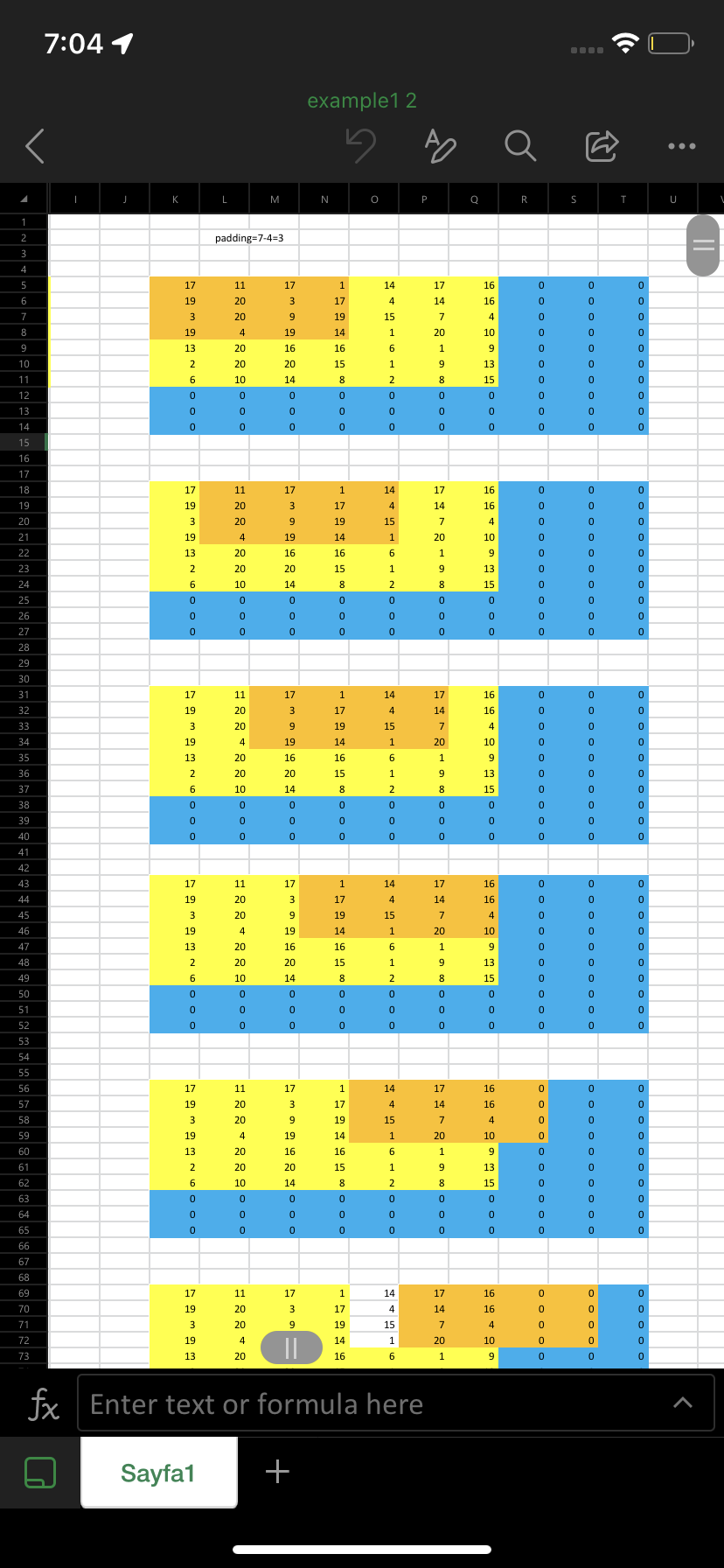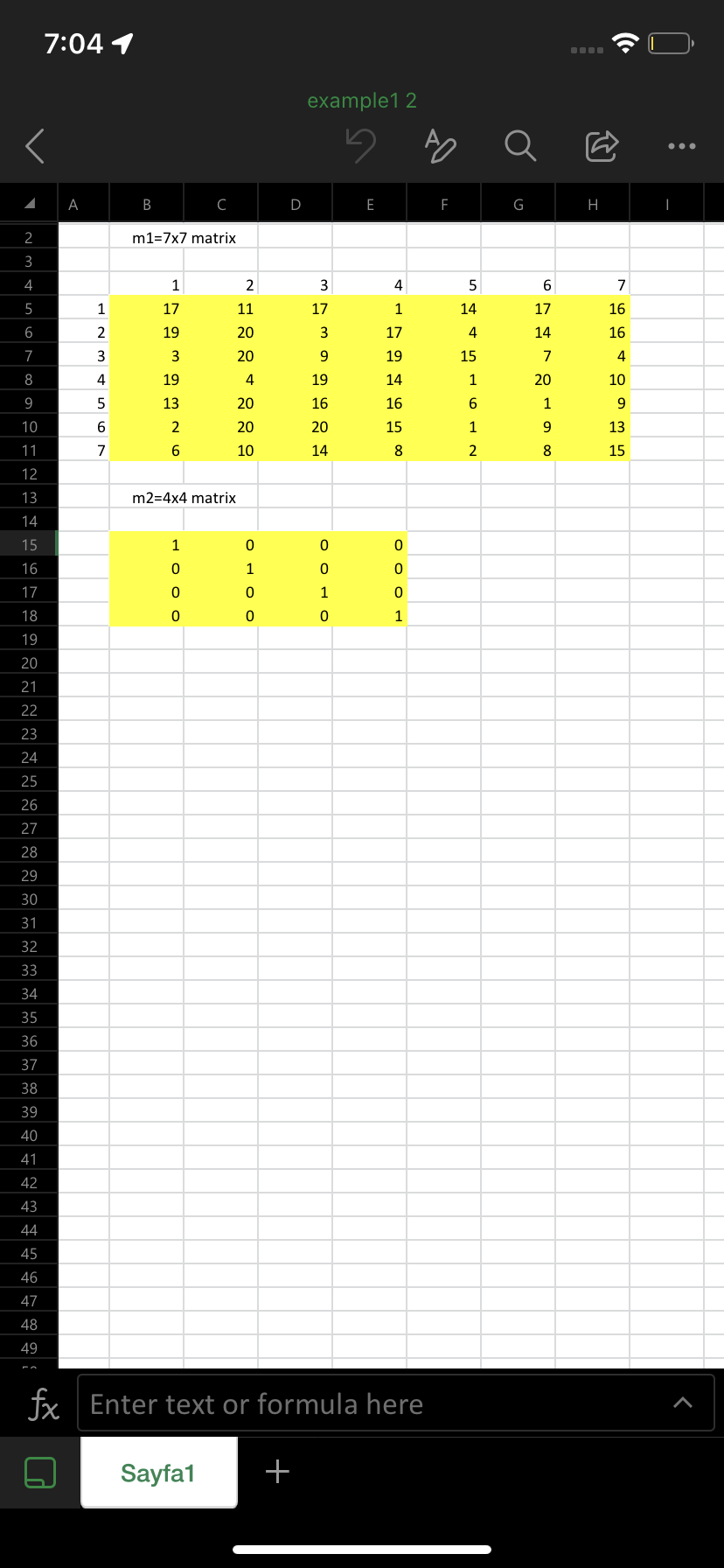# Convolution without convolution function

4 ビュー (過去 30 日間)
Randa 2022 年 11 月 30 日
コメント済み: Rena Berman 2022 年 12 月 5 日
<<>>
So I have two square matrices of different sizes. I want to multiply each element of each matrix, sum them, then place the results(column by column)in another output matrix with the same size of the bigger matrix. In order to do that, I have to pad the bigger matrix and incorporate a for loop for the smaller matrix inside the bigger matrix where is shifts by one every iteration and performs the calculations. I also have to incorporate another for loop for the bigger matrix where I can perform those calculations(multiplying then summing)but my code doesn’t work for every size:
function homework M1=rand(7) M2=eye(4)
n=size(M1)-size(M2);%subtract size M2 from size M1 to get the right output padding size A=padarray(M1,n,"post"); %padding M1 with the padded size Output=zeros(size(M1)); %output matrix,set it first to zero
for i=1:size(A,1) for j=1:size(A,2) temp=A(i:i+n,j:j+n).*M2; Output(i,j)=sum(temp(:)); end end display(Output) end##### 1 件のコメント表示 なし非表示 なし
Rena Berman 2022 年 12 月 5 日

サインインしてコメントする。

### 回答 (1 件)

Image Analyst 2022 年 11 月 30 日
I have a manual convolution demo. See attached, and use it if you want.

サインインしてコメントする。

### カテゴリ

Find more on Loops and Conditional Statements in Help Center and File Exchange

### Community Treasure Hunt

Find the treasures in MATLAB Central and discover how the community can help you!

Start Hunting!